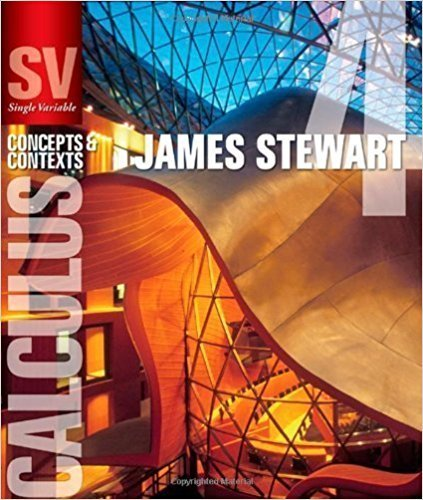×
×

# Solutions for Chapter 8.3: THE INTEGRAL AND COMPARISON TESTS; ESTIMATING SUMS## Full solutions for Single Variable Calculus: Concepts and Contexts (Stewart's Calculus Series) | 4th Edition

ISBN: 9780495559726Solutions for Chapter 8.3: THE INTEGRAL AND COMPARISON TESTS; ESTIMATING SUMS

Solutions for Chapter 8.3
4 5 0 289 Reviews
21
2
##### ISBN: 9780495559726

Single Variable Calculus: Concepts and Contexts (Stewart's Calculus Series) was written by and is associated to the ISBN: 9780495559726. This textbook survival guide was created for the textbook: Single Variable Calculus: Concepts and Contexts (Stewart's Calculus Series), edition: 4. Chapter 8.3: THE INTEGRAL AND COMPARISON TESTS; ESTIMATING SUMS includes 46 full step-by-step solutions. Since 46 problems in chapter 8.3: THE INTEGRAL AND COMPARISON TESTS; ESTIMATING SUMS have been answered, more than 20595 students have viewed full step-by-step solutions from this chapter. This expansive textbook survival guide covers the following chapters and their solutions.

Key Calculus Terms and definitions covered in this textbook
• Absolute minimum

A value ƒ(c) is an absolute minimum value of ƒ if ƒ(c) ? ƒ(x)for all x in the domain of ƒ.

P(A or B) = P(A) + P(B) - P(A and B). If A and B are mutually exclusive events, then P(A or B) = P(A) + P(B)

If u < v , then u + w < v + w

• Ambiguous case

The case in which two sides and a nonincluded angle can determine two different triangles

• Component form of a vector

If a vector’s representative in standard position has a terminal point (a,b) (or (a, b, c)) , then (a,b) (or (a, b, c)) is the component form of the vector, and a and b are the horizontal and vertical components of the vector (or a, b, and c are the x-, y-, and z-components of the vector, respectively)

• Elimination method

A method of solving a system of linear equations

• Endpoint of an interval

A real number that represents one “end” of an interval.

• Factor

In algebra, a quantity being multiplied in a product. In statistics, a potential explanatory variable under study in an experiment, .

• Fitting a line or curve to data

Finding a line or curve that comes close to passing through all the points in a scatter plot.

• Inverse cosine function

The function y = cos-1 x

• Inverse cotangent function

The function y = cot-1 x

• Measure of an angle

The number of degrees or radians in an angle

• Minor axis

The perpendicular bisector of the major axis of an ellipse with endpoints on the ellipse.

• Multiplicative inverse of a matrix

See Inverse of a matrix

• NDER ƒ(a)

See Numerical derivative of ƒ at x = a.

• Power-reducing identity

A trigonometric identity that reduces the power to which the trigonometric functions are raised.

• Range screen

See Viewing window.

• Resolving a vector

Finding the horizontal and vertical components of a vector.

A graph in which (x, -y) is on the graph whenever (x, y) is; or a graph in which (r, -?) or (-r, ?, -?) is on the graph whenever (r, ?) is